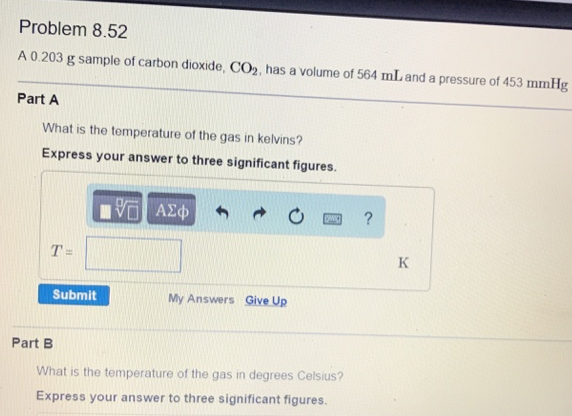# Problem: A 0.203 g sample of carbon dioxide, CO2, has a volume of 564 mL and a pressure of 453 mmHg.What is the temperature of the gas in kelvins? Express your answer to three significant figures.What is the temperature of the gas in degrees Celsius? Express your answer to three significant figures.

###### FREE Expert Solution
80% (400 ratings)###### Problem Details

A 0.203 g sample of carbon dioxide, CO2, has a volume of 564 mL and a pressure of 453 mmHg.

What is the temperature of the gas in kelvins? Express your answer to three significant figures.

What is the temperature of the gas in degrees Celsius? Express your answer to three significant figures.